Request a Tool

Combined Gas Law Calculator

Combination of three gas laws, which are boyle's law, charles's law and gay lussac's

Output

Initial Volume (V1)
0

Formula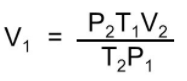• V1 = Initial Volume
• P1 = Initial Pressure
• T1 = Initial Temperature
• V2 = Final Volume
• P2 = Final Volume
• T2 = Final Volume

Defination / Uses

Boyle's Law, Charles' Law, and Gay-Law Lussac's are integrated in the combined gas law. The ratio of the product of pressure and volume to the absolute temperature of a gas is equal to a constant, according to this formula. The ideal gas law is determined by adding Avogadro's law to the combined gas law. The combined gas law, unlike the named gas laws, does not have an actual discoverer. When everything except temperature, pressure, and volume are kept constant, it is just a mix of the other gas laws.

Output

Initial Pressure (P1)
0

Formula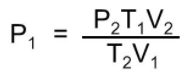• V1 = Initial Volume
• P1 = Initial Pressure
• T1 = Initial Temperature
• V2 = Final Volume
• P2 = Final Volume
• T2 = Final Volume

Defination / Uses

Boyle's Law, Charles' Law, and Gay-Law Lussac's are integrated in the combined gas law. The ratio of the product of pressure and volume to the absolute temperature of a gas is equal to a constant, according to this formula. The ideal gas law is determined by adding Avogadro's law to the combined gas law. The combined gas law, unlike the named gas laws, does not have an actual discoverer. When everything except temperature, pressure, and volume are kept constant, it is just a mix of the other gas laws.

Output

Intial Temperture (T1)
0

Formula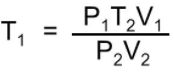• V1 = Initial Volume
• P1 = Initial Pressure
• T1 = Initial Temperature
• V2 = Final Volume
• P2 = Final Volume
• T2 = Final Volume

Defination / Uses

Boyle's Law, Charles' Law, and Gay-Law Lussac's are integrated in the combined gas law. The ratio of the product of pressure and volume to the absolute temperature of a gas is equal to a constant, according to this formula. The ideal gas law is determined by adding Avogadro's law to the combined gas law. The combined gas law, unlike the named gas laws, does not have an actual discoverer. When everything except temperature, pressure, and volume are kept constant, it is just a mix of the other gas laws.

Output

Final Volume (V2)
0

Formula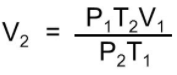• V1 = Initial Volume
• P1 = Initial Pressure
• T1 = Initial Temperature
• V2 = Final Volume
• P2 = Final Volume
• T2 = Final Volume

Defination / Uses

Boyle's Law, Charles' Law, and Gay-Law Lussac's are integrated in the combined gas law. The ratio of the product of pressure and volume to the absolute temperature of a gas is equal to a constant, according to this formula. The ideal gas law is determined by adding Avogadro's law to the combined gas law. The combined gas law, unlike the named gas laws, does not have an actual discoverer. When everything except temperature, pressure, and volume are kept constant, it is just a mix of the other gas laws.

Output

Final Pressure (V2)
0

Formula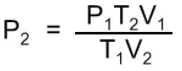• V1 = Initial Volume
• P1 = Initial Pressure
• T1 = Initial Temperature
• V2 = Final Volume
• P2 = Final Volume
• T2 = Final Volume

Defination / Uses

Boyle's Law, Charles' Law, and Gay-Law Lussac's are integrated in the combined gas law. The ratio of the product of pressure and volume to the absolute temperature of a gas is equal to a constant, according to this formula. The ideal gas law is determined by adding Avogadro's law to the combined gas law. The combined gas law, unlike the named gas laws, does not have an actual discoverer. When everything except temperature, pressure, and volume are kept constant, it is just a mix of the other gas laws.

Output

Final Temperature (T2)
0

Formula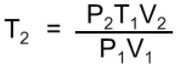• V1 = Initial Volume
• P1 = Initial Pressure
• T1 = Initial Temperature
• V2 = Final Volume
• P2 = Final Volume
• T2 = Final Volume

Defination / Uses

Boyle's Law, Charles' Law, and Gay-Law Lussac's are integrated in the combined gas law. The ratio of the product of pressure and volume to the absolute temperature of a gas is equal to a constant, according to this formula. The ideal gas law is determined by adding Avogadro's law to the combined gas law. The combined gas law, unlike the named gas laws, does not have an actual discoverer. When everything except temperature, pressure, and volume are kept constant, it is just a mix of the other gas laws.

Each of these rules scientifically connects one thermodynamic variable to another while preserving everything else constant. As long as pressure is constant, Charles' law asserts that volume and temperature are exactly proportionate. At constant temperature, Boyle's law states that pressure and volume are inversely proportional.
Finally, Gay-Lussac's law introduces a direct proportionality between temperature and pressure as long as it is at a constant volume. The inter-dependence of these variables is shown in the combined gas law, which clearly states that: The ratio between the pressure-volume product and the temperature of a system remains constant. Use the upper given formula for manual calculations. No sign-up, registration OR captcha is required to use this tool.